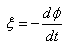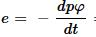Courses

# Test: Faraday’S Laws Of Induction

## 10 Questions MCQ Test Physics Class 12 | Test: Faraday’S Laws Of Induction

Description
This mock test of Test: Faraday’S Laws Of Induction for JEE helps you for every JEE entrance exam. This contains 10 Multiple Choice Questions for JEE Test: Faraday’S Laws Of Induction (mcq) to study with solutions a complete question bank. The solved questions answers in this Test: Faraday’S Laws Of Induction quiz give you a good mix of easy questions and tough questions. JEE students definitely take this Test: Faraday’S Laws Of Induction exercise for a better result in the exam. You can find other Test: Faraday’S Laws Of Induction extra questions, long questions & short questions for JEE on EduRev as well by searching above.
QUESTION: 1

### A magnet is moved towards the coil (i) quickly, (ii) slowly, the induced emf is​

Solution:

When a magnet is moved towards the coil quickly, the rate of change of flux is larger than that if the magnetic field is moved slowly, thus larger emf is induced due to quick movement of the coil.

QUESTION: 2

### The magnetic flux linked with a coil is changed from 1 Wb to 0.1 Wb in 0.1 second. The induced emf is​

Solution:

Change n in magnetic flux Δϕ=0.1−1=−0.9 Weber
Time taken  Δt=0.1 sec
So, emf induced in the coil  E=− Δϕ​/Δt
⟹ E=− (−0.9)​/ 0.1=9 Volts
Hence option C is the correct answer.

QUESTION: 3

### The principle of electromagnetic induction is used in which of the following phenomenon

Solution:

In hydroelectricity the power of a turbine depends on the size of the water drop and the quantity of water flowing through the turbine. The water propels the turbine, which in turn powers an electric generator, which produces electricity under the principle of electromagnetic induction.

QUESTION: 4

Which of the following law’s gives the direction of induced emf?

Solution:

Lenz’s law is used for determining the direction of induced current.
Lenz’s law of electromagnetic induction states that the direction of induced current in a given magnetic field is such that it opposes the induced change by changing the magnetic field.
Following is the formula of Lenz’s law:
ϵ=−N (∂ϕB/∂t)
Where,

• ε is the induced emf
• ∂ΦB is the change in magnetic flux
• N is the number of turns in the coil

Lenz’s law finds application in electromagnetic braking and in electric generators

QUESTION: 5

In the expression, what is the significance of negative sign?

Solution:

The negative sign signifies that the induced emf always opposes any change in the magnetic flux.

QUESTION: 6

Magnetic flux φ in Weber in closed circuit of resistance 15 ohm varies with time t sec as φ = 2t² – 3t + 1. The magnitude of induced emf at t = 0.25 sec is

Solution:= 4t-3
At t = 0.25s
e= 2V

QUESTION: 7

If the North Pole of the bar magnet is introduced into a coil at the end A which of the following statements correctly depicts the phenomenon taking place.

Solution:

According to Lenz’s law the end A becomes the North Pole to oppose the motion of the magnet.
Hence, option B is correct.

QUESTION: 8

In which of the following cases with a bar magnet and the coil no induced emf is induced.

Solution:

This is because induced emf directly depends on relative motion between the magnet and coil, but if there is no relative motion between them, there will be no emf induced.

QUESTION: 9

The role of inductance is equivalent to:

Solution:

Self induction is that phenomenon in which a change in electric current in a coil produces an induced emf in the coil itself.
Now, it is also known as inertia of electricity as for if we were to change electric current through a current carrying coil it will tend to oppose any further change in its emf. This is similar to inertial behavior in mechanics where bodies in either rest or motion tend to oppose any change in their state. Here mass is the inertial property analogous to self inductance.

QUESTION: 10

A coil of 100 turns is pulled in 0.04 sec. between the poles of a magnet, if its flux changes from 40 x 10-6 Wb per turn to 10-5 Wb per turn, then the average emf induced in the coil is​

Solution:

Emf = - N(∆magnetic flux)/∆t
N=100turns
∆magnetic flux= -30×10-6Wb
∆t = 0.04sec
Emf = -(100turns)(-30×10-6)/(0.04sec)
Emf = 0.075V